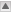Logic in AMPL

Relational Operators

Relational operators are used to compare two expressions. They are most commonly used in constraints, but not exclusively. The relational operators are:

ExpressionMeaning
> Greater than
>= Greater than or equal to
>= Equal to
< Less than
<= Less than or equal to
<> Not equal to

Logical Operators

Logical operators are used to combine logical expressions. They are most commonly used in conditional statements, conditional structures and conditional loops. The logical operators are not, and and or. They are used as shown in the table below:

Expression Result
not <expression> True if <expression> is false, false if <expression> is true
<e1> and <e2> True if <e1> and <e2> are both true, otherwise false
<e1> or <e2> True if either <e1> or <e2> are true, otherwise false

Logical Expressions

Logical expressions are expressions that will evaluate to either true or false. Logical expressions are usually defined in terms of the relational operators:

Lower[r] <= sum {i in INGREDIENTS} Contributes[r, i] * Amount[i]

sum {s in SURFBOARDS} Recipe[m, s] * Production[s] <= Supply[m];

However, there are some special logical expressions in AMPL for use with sets:

1. <e> in <SET> is true if <e> is a member of <SET>;
2. <e> not in <SET> is false if <e> is a member of <SET>;
3. exists {<e> in <SET>} <expression> is true if some <e> in <SET> has <expression> being true;
4. forall {<e> in <SET>} <expression> is true if all <e> in <SET> have <expression> being true;
5. <SUBSET> within <SET> is true if all the elements in <SUBSET> are in <SET>;
6. <SUBSET> not within <SET> is true if some element in <SUBSET> is not in <SET>.

Logical expressions can be built up from other logical expressions, binary parameters and logical operators.

Conditional Expressions

A conditional expression is very much like the IF function in Microsoft Excel:

param ifvalue := if <some logical expression> then
<a value>
[else
<another value>];

If the logical expression is true then ifvalue will be set to <a value>, otherwise it is set to 0 (by default) or, if the else part of the expression is present, <another value>. Note that if the else keyword is present, then no ; needs to be included after <a value>.

Conditional Structures

A conditional structure is the same as the classical if_-_then_-_else statement in programming languages like MATLAB, Fortran, Visual Basic and C++:

if <logical expression> then
<a statement>;
[else
<another statement>;]

Note here that even if the else keyword is present you need to end <a statement> with ;. If you want to include more than one statement within the conditional structures you can use { and } to enclose your statements:

if <logical expression> then
{
<some statements>
}
[else
{
<some other statements>
}]

Binary Parameters

In AMPL we can create binary parameters by using the binary keyword in the parameter declaration:

param stillSearching binary;

Binary parameters are used in a similar way to boolean variables (in Matlab, C, etc) and logical variables (Fortran). If a binary parameter has the value 0 this is equivalent to false, and 1 is equivalent to true. Binary parameters can be used with conditional expressions to hold a true/false result from a logical expression:

param isGreater binary;

let isGreater := if 4 > 5 then 1 else 0; # isGreater = 0 (false)
let isGreater := if 6 > 5 then 1; # else 0 is the default, isGreater = 1 (true)
so the syntax is
let <binary parameter> := if <expression> then 1;

You can also set binary parameters within conditional structures

binary <binary parameter>;

if <expression> then
let <binary parameter> := 1;
else
let <binary parameter> := 0;

Binary parameters may be used in logical expressions or as the condition in a conditional statement or conditional structure. They are very useful for building complex conditional statements or structures:

Some example from depth first searching or column generation
Coming soon! and controlling conditional loops:
Some example from depth first searching or column generation
Coming soon!

-- TWikiAdminGroup - 18 Mar 2008

This topic: OpsRes > WebHome > AMPLGuide > AMPLSyntax > LogicInAMPL
Topic revision: r6 - 2008-04-06 - MichaelOSullivan

Copyright © 2008-2022 by the contributing authors. All material on this collaboration platform is the property of the contributing authors.
Ideas, requests, problems regarding TWiki? Send feedback